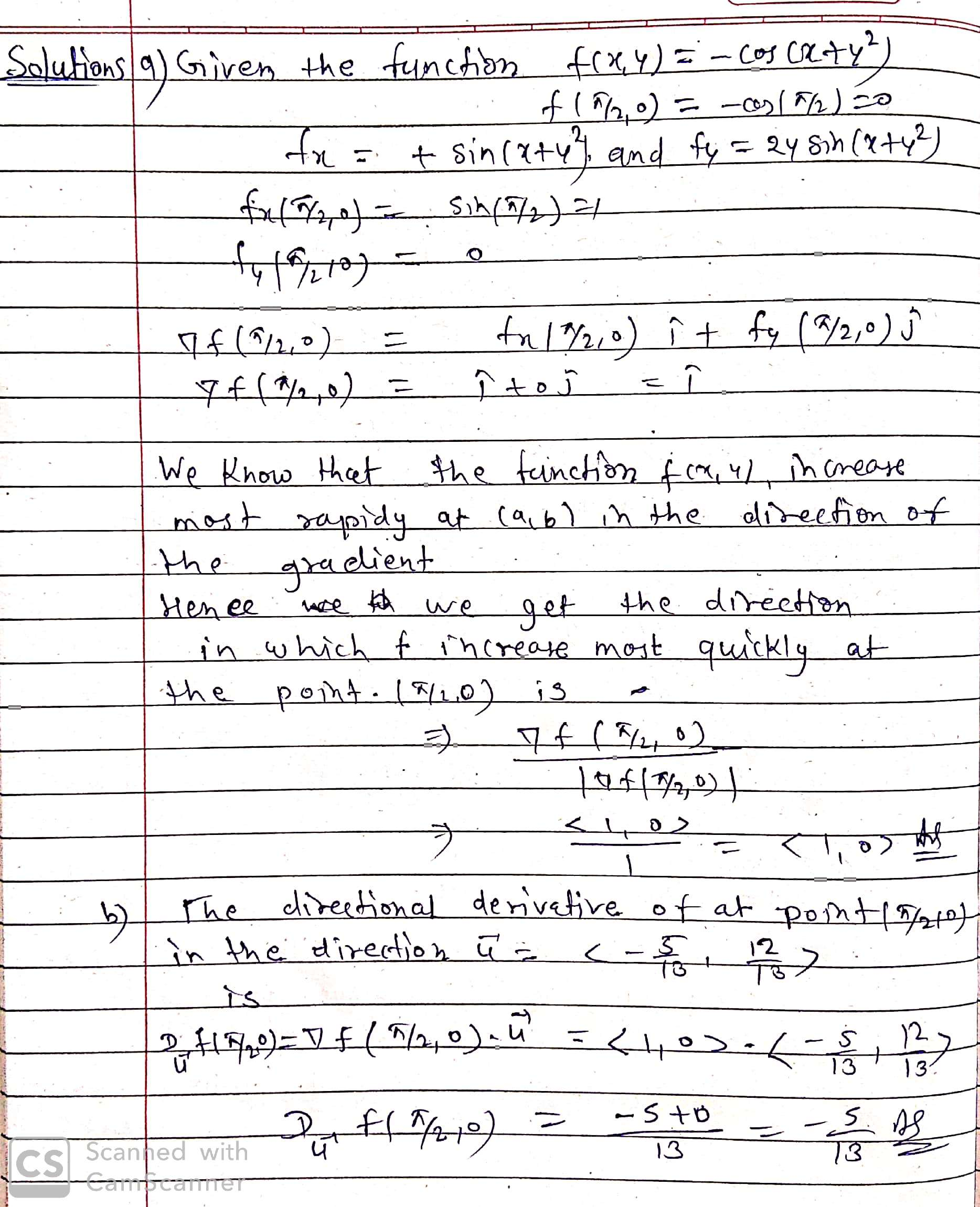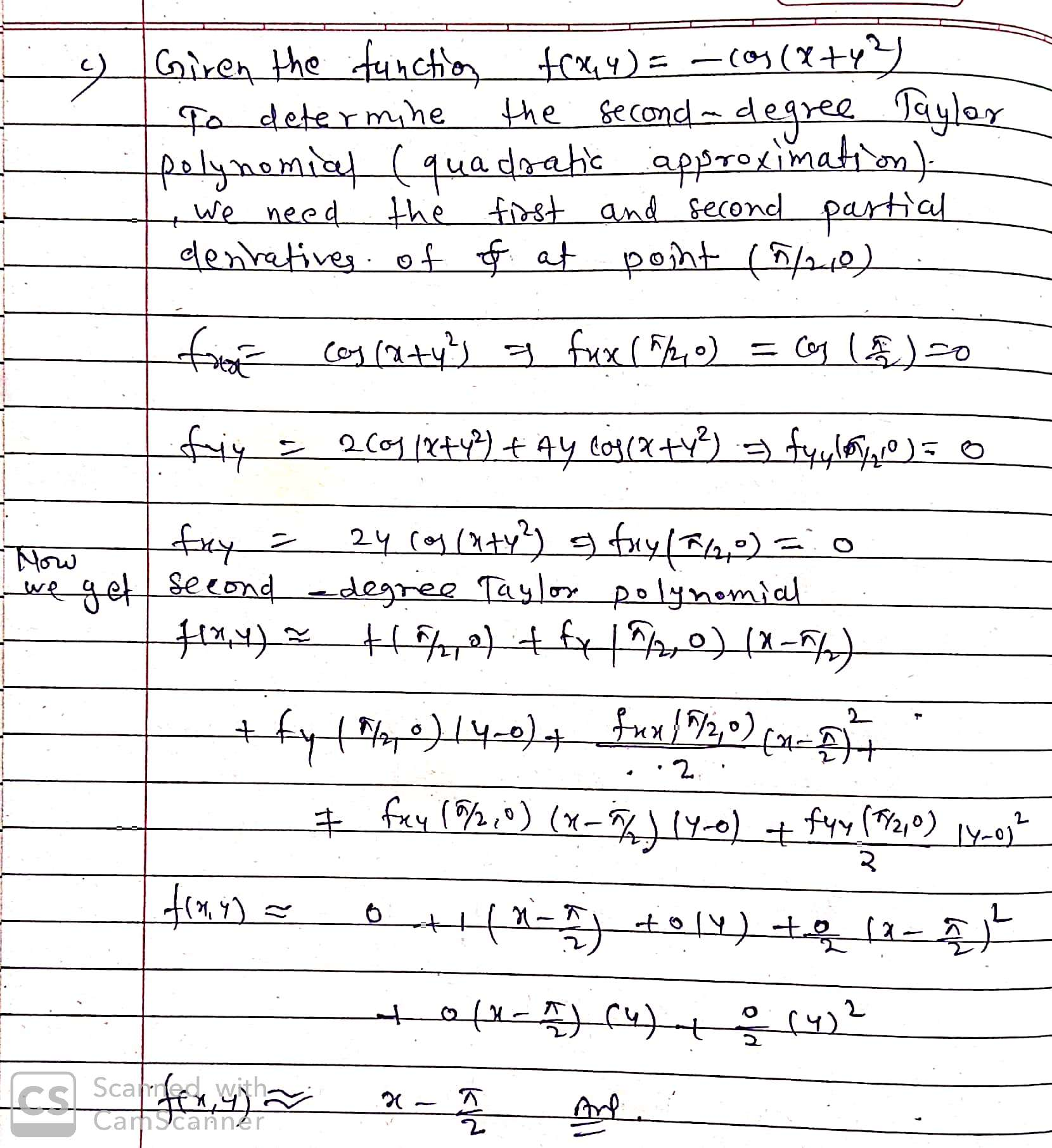In: Math

# Let f(x, y) = − cos(x + y2 ) and let a be the point a...

Let f(x, y) = − cos(x + y2 ) and let a be the point a = ( π/2, 0).

(a) Find the direction in which f increases most quickly at the point a.

(b) Find the directional derivative Duf(a) of f at a in the direction u = (−5/13 , 12/13) .

(c) Use Taylor’s formula to calculate a quadratic approximation to f at a.

## Solutions

##### Expert Solution## Related Solutions

##### f(r,?) f(x,y) r(cos(?)) = x r(cos(2?)) = ? r(cos(3?)) = x3-3xy2/x2+y2 r(cos(4?)) = ? r(cos(5?)) =...
f(r,?) f(x,y) r(cos(?)) = x r(cos(2?)) = ? r(cos(3?)) = x3-3xy2/x2+y2 r(cos(4?)) = ? r(cos(5?)) = ? Please complete this table. I am having trouble converting functions from polar to cartesian in the three dimensional plane. I understand that x=rcos(?) and y=rsin(?) and r2 = x2 + y2 , but I am having trouble understanding how to apply these functions.
##### Let F (x, y) = y sin x i – cos x j, where C is...
Let F (x, y) = y sin x i – cos x j, where C is the line segment from (π/2,0) to (π, 1). Then C F•dr is A 1 B 2 C 5/2 D 3 E 7/2
##### Let f(x, y) = x2y(2 − x + y2)5 − 4x2(1 + x + y)7 +...
Let f(x, y) = x2y(2 − x + y2)5 − 4x2(1 + x + y)7 + x3y2(1 − 3x − y)8. Find the coefficient of x5y3 in the expansion of f(x, y)
##### Calculate the second-order Taylor approximation to f(x, y) = 3 cos(x) sin(y) at the point (pi,...
Calculate the second-order Taylor approximation to f(x, y) = 3 cos(x) sin(y) at the point (pi, pi/2)
##### Let f(x,y) be a scalar function, and let F(x,y,z) be a vector field. Only one of...
Let f(x,y) be a scalar function, and let F(x,y,z) be a vector field. Only one of the following expressions is meaningful. Which one? a) grad f x div F b) div(curl(grad f)) c) div(div F) d) curl(div(grad f)) e) grad(curl F)
##### Let f ( x , y ) = x^ 2 + y ^3 + sin ⁡...
Let f ( x , y ) = x^ 2 + y ^3 + sin ⁡ ( x ^2 + y ^3 ). Determine the line integral of f ( x , y ) with respect to arc length over the unit circle centered at the origin (0, 0).
##### The value of f depends on two independent variables x and y as defined below: f(x,y)=x2+y2−x+3cos(x)sin(y)...
The value of f depends on two independent variables x and y as defined below: f(x,y)=x2+y2−x+3cos(x)sin(y) Function f has a minimum in the neighborhood of the origin (i.e. [0 0]). Find x and y which minimize f. Note: You are not allowed to use MATLAB built-in functions for optimization. Follow the logic of the derivative test: The minimum of f occurs where: ∂f∂x=0∂f∂y=0
##### Let f(x, y, z) = 3x^(2)y + 4e^(2x+y) − 6 cos(xyz) (a) Find fx (b) Find...
Let f(x, y, z) = 3x^(2)y + 4e^(2x+y) − 6 cos(xyz) (a) Find fx (b) Find fy (c) Find fz (d) Find fxy (e) Find fyx (f) Find fxzx (g) Find fzzz
##### a. (5 Marks) 1 1 cos(x)cos(y) = -cos(x-y) + -cos(x + y) 1 l sin(x)sin(y) =...
a. 1 1 cos(x)cos(y) = -cos(x-y) + -cos(x + y) 1 l sin(x)sin(y) = -cos(x-y)--cos(x+ y) 1 l sin(x)cos(y) =—sin(x-y) +-sin(x + y) A DSB-FC (double sideband-full carrier) signal s(t) is given by, s(t) = n cos(2rr/cf)+ cos(2«-/mt)cos(2«-fct) What is the numeric value for the AM index of modulation, m, fors(f) ?
##### Let f(x, y) = 5x 2y − 3x2  + 2y3 + 3xy, P be the point (1,...
Let f(x, y) = 5x 2y − 3x2  + 2y3 + 3xy, P be the point (1, −2) and a = <3, −5>. This problem has five parts. (a) [5 pts.] Find the first partial derivatives of f(x, y). (b) [5 pts.] Find all of the second-order partial derivatives of f(x, y). (c) [5 pts.] Find an equation of the tangent plane to f(x, y) at P. (d) [5 pts.] Find ∇f. (This is still part of number 8) (e)Find the...